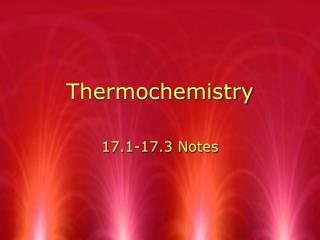DownloadDownload PresentationThermochemistry

# Thermochemistry

Download Presentation## Thermochemistry

- - - - - - - - - - - - - - - - - - - - - - - - - - - E N D - - - - - - - - - - - - - - - - - - - - - - - - - - -
##### Presentation Transcript

1. Thermochemistry 17.1-17.3 Notes

2. Energy • Energy = the ability to do work or produce temperature change. • Chemical Potential Energy is the energy stored in the chemical bonds of a substance

3. Heat • Heat is the energy that flows from a warmer object to a cooler object. • Heat energy is represented by the symbol q. • Transfer of heat causes change in temperature.

4. Measuring Heat • 1 calorie = amount of energy required to raise 1 gram of water by 1 °C. • 1 Calorie (big C) = 1000 calories. (a.k.a. kilocalorie) • The SI unit for heat is the Joule (J) • 1 cal = 4.184 J

5. sToP & tHinK • How many joules are in 2 calories?

6. Specific Heat • The specific heat of any substance is the amount of heat required to raise 1 gram of the substance 1 degree Celsius. • units are J/(g* °C) • represented by the symbol c

7. sToP & tHinK • You have two 20 g samples of Pb and Al. Which one will require more energy to change the temperature by 10 °C? How did you reach your conclusion? • Lead 0.130 J/(g x °C) • Aluminum 0.903 J/(g x °C)

8. Energy in Temperature Change • q=mcΔT • Heat absorbed/released=q (+ or -) • Specific Heat of the sample=c (+) • Mass of the sample in grams=m (+) • Change in temperature: (+ or -) • Tfinal-Tinitial = ΔT

9. sToP & tHinK • How much energy is needed to raise a 20 gram sample of water by 20 °C? The specific heat of water is 4.184 J/g °C. • The temperature of a 10 g sample of iron changed from 25 °C to 50.0 °C and gained 112.5 J of heat. What is the specific heat ( c) of the sample? • How much energy is released when a 10 g sample of ice goes from -5 to -10 °C? The specific heat of ice is 2.03 J/g °C

10. Heat in Systems… • Thermochemistry = study of heat involved in chemical reactions or phase change • system - contains the object of study • surroundings - everything other than the system

11. Enthalpy • Enthalpy, the symbol H, is the heat content of a system at constant pressure. • The change in enthalpy for a reaction is called the enthalpy (heat) of reaction, ∆Hrxn • ∆Hrxn = H products - H reactants

12. Heat and Chemical Reactions • thermochemical equation = a balanced equation with phase and enthalpy, ΔH. • example: • 4 Fe(s) + 3O2(g) -> 2Fe2O3(s) ΔH = -1625kJ

13. Exothermic Reactions • Exothermic reactions have a negative sign of ΔHrxn • H products < H reactants • energy is released

14. Endothermic Reactions • Endothermic reactions have a positive sign of ΔHrxn • H products > H reactants • energy is absorbed

15. sToP & tHinK The Hproducts equals 2500 kJ and the Hreactants equals 2000 kJ. 1. What is the Hrxn? 2. Is this reaction endothermic or exothermic? 3. Draw what the energy diagram might look like for this reaction.

16. q=mc ∆T shows energy released/absorbed during temperature changes q=m ∆Hfus or q=m ∆Hvap shows energy released/absorbed during phase changes Two equations for heating curves…

17. Heat & Phase Changes • Latent Heat of Fusion = amount of heat needed to melt one mole or gram of substance • ΔHfus = - ΔHsolid • Latent Heat of Vaporization = amount of heat needed to vaporize one mole or gram of substance • ΔHvap = - ΔHcond

18. Solid to liquid to gas - endothermic, +q , gains energy Gas to liquid to solid - exothermic, -q, releases energy

19. sToP & tHinK Ammonia, NH3, has a latent heat of vaporization (ΔHvap) of 23.3 kJ/mol. 1. How much energy would be required to convert 5 moles of ammonia from liquid to gas? 2. How much energy would be released when converting 25 moles of ammonia from gas to liquid?

20. Combining Equations • If a problem includes both temperature change and phase change, use both equations. • Example: How much energy is needed to heat 100 g of water from 20 C to 120 C?